# Optical mammography (Detecting and monitoring the breast cancer)

### Introduction

The breast cancer incidence rate has been increasing in Japanese women. A measurement technology for diagnosing and monitoring responses to the treatment is important to improve the QOL (Quality of Life) of breast cancer patients. The near infrared spectroscopy (NIRS), which is a noninvasive measurement technique to quantify the absorption and scattering coefficients, hemoglobin concentration, and tissue oxygen saturation in the biological tissue, is expected to be useful for detecting change in those parameters due to growth or shrinkage of the breast cancer. The optical mammography is a NIRS system designed for breast measurement and will provide earlier detection and easier monitoring of the breast cancer.

### Time-resolved spectroscopy

The light used for NIRS measurement is near-infrared light in the wavelength range 700 nm to 1100 nm which is relatively easier to pass through the biological tissue compared to other wavelength. The light by which biological tissue is irradiated is mainly absorbed by hemoglobin, water and lipid. The concentration of the oxy-hemoglobin (O2Hb)and deoxy-hemoglobin(HHb)can be quantified based on their specific absorption characteristics. The tissue oxygen saturation (StO2) is obtained from the ratio of O2Hb to the total hemoglobin (tHb). We use a measurement technique utilizing pulsed laser called time-resolved spectroscopy (TRS) which is expected to show better quantitativeness and reproducibility compared to techniques utilizing CW laser and intensity modulated laser.

#### The principle of the time-resolved spectroscopy

As shown in Figure 1, the biological tissue is irradiated with near-infrared pulsed laser, and the light passed through the tissue is detected. In order to obtain the absorption and scattering coefficients of the biological tissue, the temporal profile of the detected light is analyzed based on the photon diffusion theory which expresses the photon migration in the scattering media. By using Eq. (1), the $$O_2Hb$$, $$HHb$$, $$tHb$$, and $$StO_2$$ are determined from the absorption coefficients obtained for several wavelengths.

$$\mu_{a}^{\lambda}$$ = $$\varepsilon_{O_{2}Hb}^{\lambda}$$ $$C_{O_{2}Hb}$$+$$\varepsilon_{HHb}^{\lambda}$$ $$C_{HHb}$$+$$\mu_{a,BG}^{\lambda}$$  (1)

$$\varepsilon_{O_{2}Hb}^{\lambda}$$: Molar extinction coefficient of oxy-hemoglobin ($$cm^{-1}・\mu M^{-1}$$)
$$\varepsilon_{HHb}^{\lambda}$$: Molar extinction coefficient of deoxy-hemoglobin ($$cm^{-1}・\mu M^{-1}$$)
$$\mu_{a,BG}^{\lambda}$$: Absorption coefficient of background medium($$cm^{-1}$$)
$$C_{O_{2}Hb}$$($$\mu M$$): Oxy-hemoglobin concentration
$$C_{HHb}$$($$\mu M$$): Deoxy-hemoglobin concentration
$$C_{tHb}$$ = $$C_{O_{2}Hb}＋C_{HHb}$$($$\mu M$$): Total hemoglobin concentration
$$StO_2$$ ＝100$$\times\frac{C_{O2Hb}}{C_{tHb}}$$($$％$$): Tissue oxygen saturationFigure 1. Schematic of the time-resolved spectroscopy in reflectance geometry.

### Optical mammography

Figure 2 shows the optical mammography which we are aiming at developing as a compact and portable diagnostic imaging system to obtain functional image at the bedside.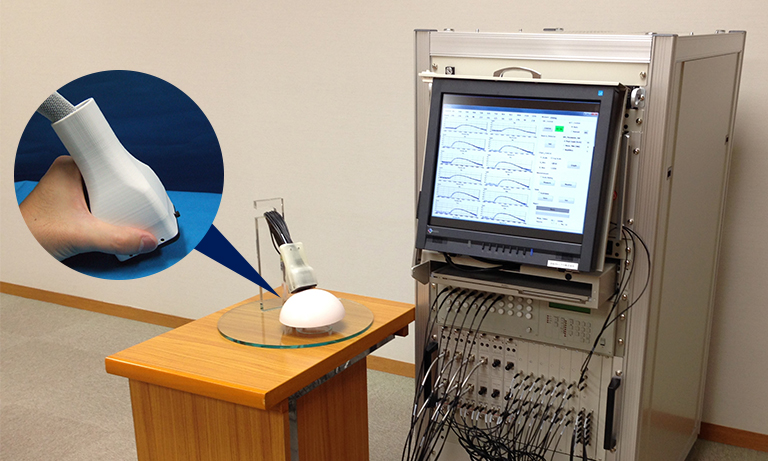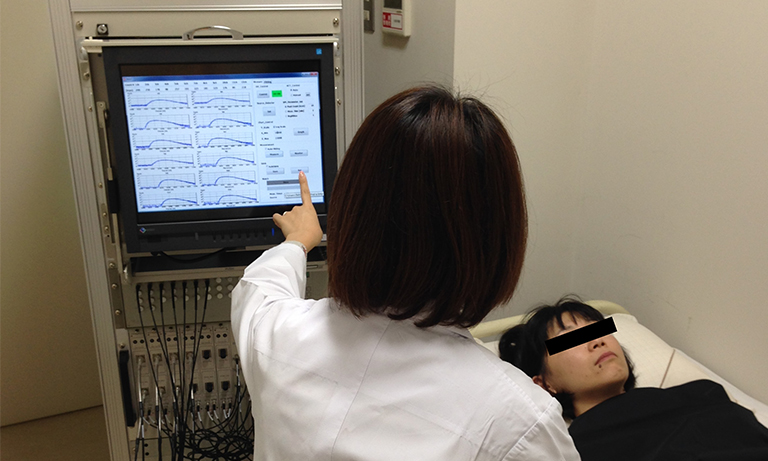Figure 2. The pictures of optical mammography. The hand-held probe (left) is used to carry out the measurement in a supine position (right).

### Preliminary clinical measurements

Preliminary clinical measurements were performed to confirm the ability of the optical mammography to measure responses during chemotherapy in a breast cancer patient, who received treatment at Hamamatsu university school of medicine. Measurement were carried out at three time points to measure responses during chemotherapy: a baseline measurement was performed before starting chemotherapy, and the second and third measurements were carried out 3 and 6 weeks after starting chemotherapy, respectively. The ultrasound images of the breast tumor shown in Figure 3 indicate that the tumor size had gradually decreased during chemotherapy. The tomographic images and plots of the maximum values within the reconstruction region of tHb concentration are shown in Figure 3. These results demonstrated that the tHb concentration was higher in the diseased breast compared with the healthy breast, and decreased during chemotherapy. In these measurements, we confirmed that the optical mammography has the potential to detect responses during chemotherapy. Measuring the hemoglobin concentration and tissue oxygen saturation is expected to be useful to detect the responses during chemotherapy and evaluate the effect of the treatment in a short time (one to 10 days).

(a)Location of tumor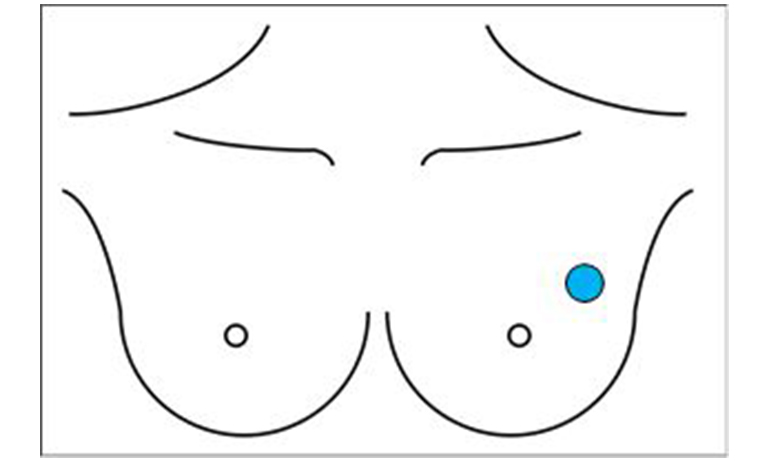Location of tumor

(b)

Baseline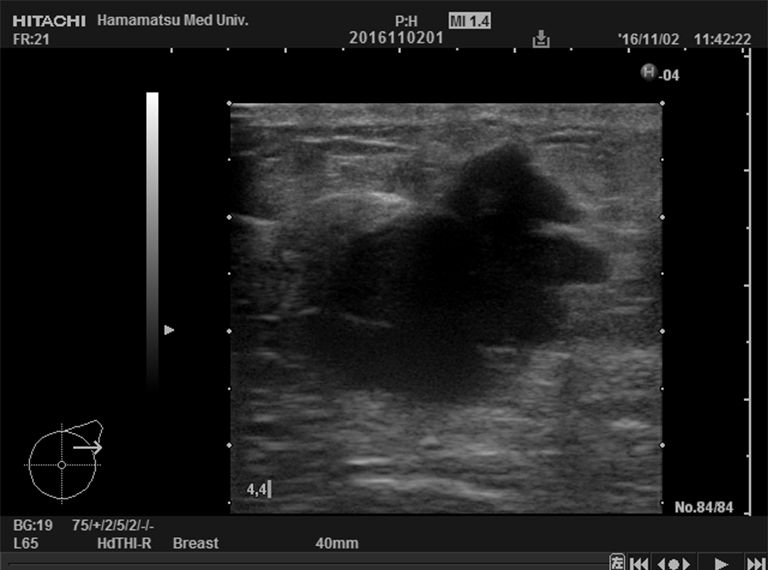26×28×21 mm

3 weeks later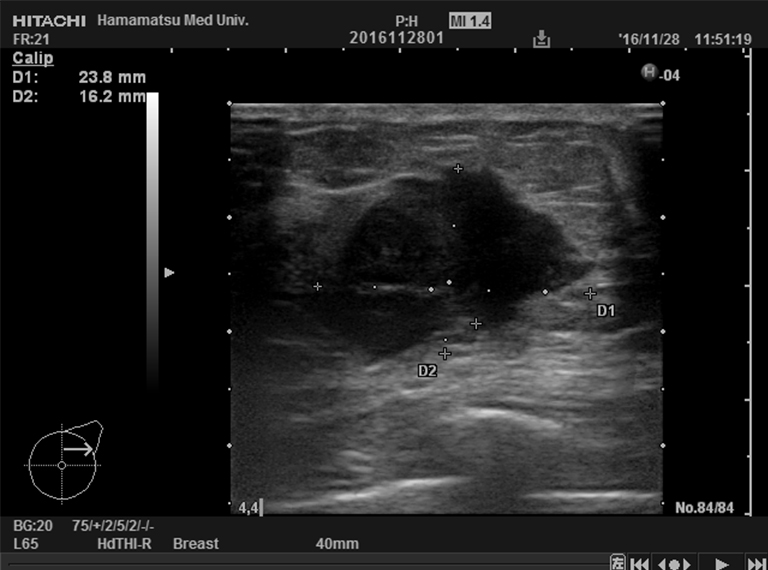26×22×17 mm

6 weeks later18×16×10 mm

(c)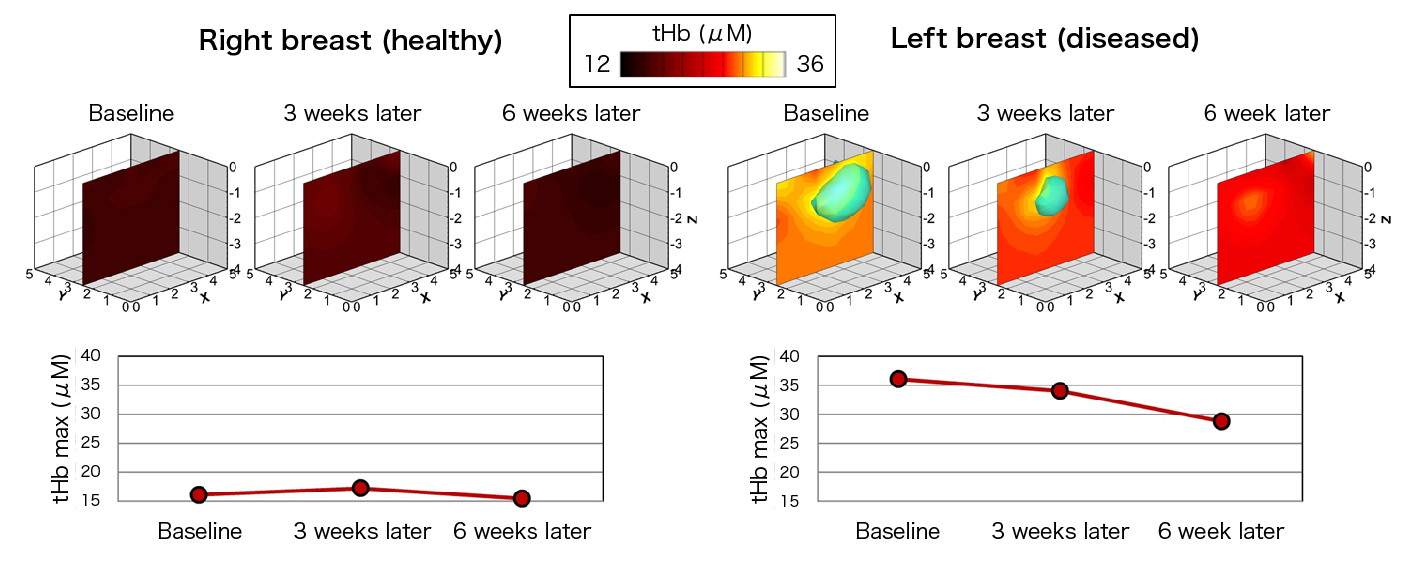Figure 3. The location of tumor (a), ultrasound images (b), and reconstructed images and plots of maximum value of total hemoglobin concentration obtained by optical mammography (c) during chemotherapy in a breast cancer patient.

### Future work

We intend to modify the measurement procedure and image reconstruction algorithm to improve the quality and quantitativeness of the reconstructed image.